(The following is copied from the readme at github.com/andyk/recursive_llm); Last updated: April 3, 2023

The idea here is to implement recursion using English as the programming language and an LLM (e.g., GPT-3.5) as the runtime.

Basically we come up with an LLM prompt which causes the model to return another slightly updated prompt. More specifically, the prompts contain state and each recursively generated prompt updates that state to be closer to an end goal (i.e., a base case).

It’s kind of like recursion in code, but instead of having a function that calls itself with a different set of arguments, there is a prompt that returns itself with specific parts updated to reflect the new arguments.

For simplicity, let’s start without a base case; here is an infinitely recursive fibonacci prompt:

You are a recursive function. Instead of being written in a programming language, you are written in English. You have variables FIB_INDEX = 2, MINUS_TWO = 0, MINUS_ONE = 1, CURR_VALUE = 1. Output this paragraph but with updated variables to compute the next step of the Fibbonaci sequence.

To “run this program” we can paste it into OpenAI playground, and click run, and then take the result and run that, etc.

In theory, because this does not specify a base case, we could stay in this loop of copying and pasting and running these successive prompts forever, each prompt representing one number in the Fibonacci sequence.

In `run_recursive_gpt.py` we automate this by writing a recursive Python function that repeatedly calls the OpenAI API with each successive prompt until the result satisfies the base case. Here’s the code from run_recursive_gpt.py:

``````def recursively_prompt_llm(prompt, n=1):
if prompt.startswith("You are a recursive function"):
prompt = openai.Completion.create(
model="text-davinci-003",
prompt=prompt,
temperature=0,
max_tokens=2048,
)["choices"]["text"].strip()
print(f"response #{n}: {prompt}\n")
recursively_prompt_llm(prompt, n + 1)

``````

And here’s what it looks like when you run it:

Note that there are two types of recursion at play here:

• First, a recursive prompt (i.e., a blob of text) that, when passed to an LLM like GPT3.5, causes some more text to be generated that itself, when passed to an LLM like GPT3.5, causes some more text to be generated, etc. etc.
• Second, a recursive Python function that first calls the model with the prompt, and then calls itself with whatever the model returned.

## Big picture goal: breaking down the problem & using memorized knowledge

The bigger picture goal here is to explore using prompts to generate new prompts, and more specifically the case where the prompts contain state and each recursively generated prompt updates that state to be closer to an end-goal.

This is partly inspired by goal-conditioned Reinforcement Learning , and also the historical AI system from CMU called General Problem Solver (GPS), which is built on an idea called means-ends analysis. Here is how GPS works at a high level: the user specifies a goal, and then GPS evaluates the difference between the current state of the world and the goal state and then tries take a step to reduce the gap, then repeat. For a high-level intro to GPS, see Patrick H. Winston’s description of it in his OCW lecture on Cognitive Architectures as part of his AI course at MIT. The ability for LLMs to break down problem into sub-steps via Chain of thought (CoT) prompting  reminded me of this part of Winston’s lecture. And so I wanted to try making a prompt that (1) contains state and (2) can be used to generate another prompt which has updated state.

To do this effectively we need to leverage the knowledge encoded into the LLM, i.e. what the LLM has learned/memorized.

The way humans do math in our heads is an interesting analog. Our brains use two types of rules that we have memorized:

1. algebraic rules for breaking the problem into sub-parts and deciding the order in which those need to be evaluated. E.g., `x+y*z = x+(y*z)`
2. atomic rules. E.g., `3*2 = 6`

Our brains use these rules to repeatedly substitue parts of the math problem until we arrive at an irreducible answer (e.g., a scalar).

• `2+3*2 # the initial math statement`
• `2 + (3*2) # use memorized rules of algebra to break the problem into two sub-problems`
• `2+6 # use memorized rule to replace 3*2 with 6`
• `8 # use memorized rule to substitue 2+6 with 8`
• `Realize that we have reached an irreducible statement so stop`

We know LLMs have memorized both types of rules: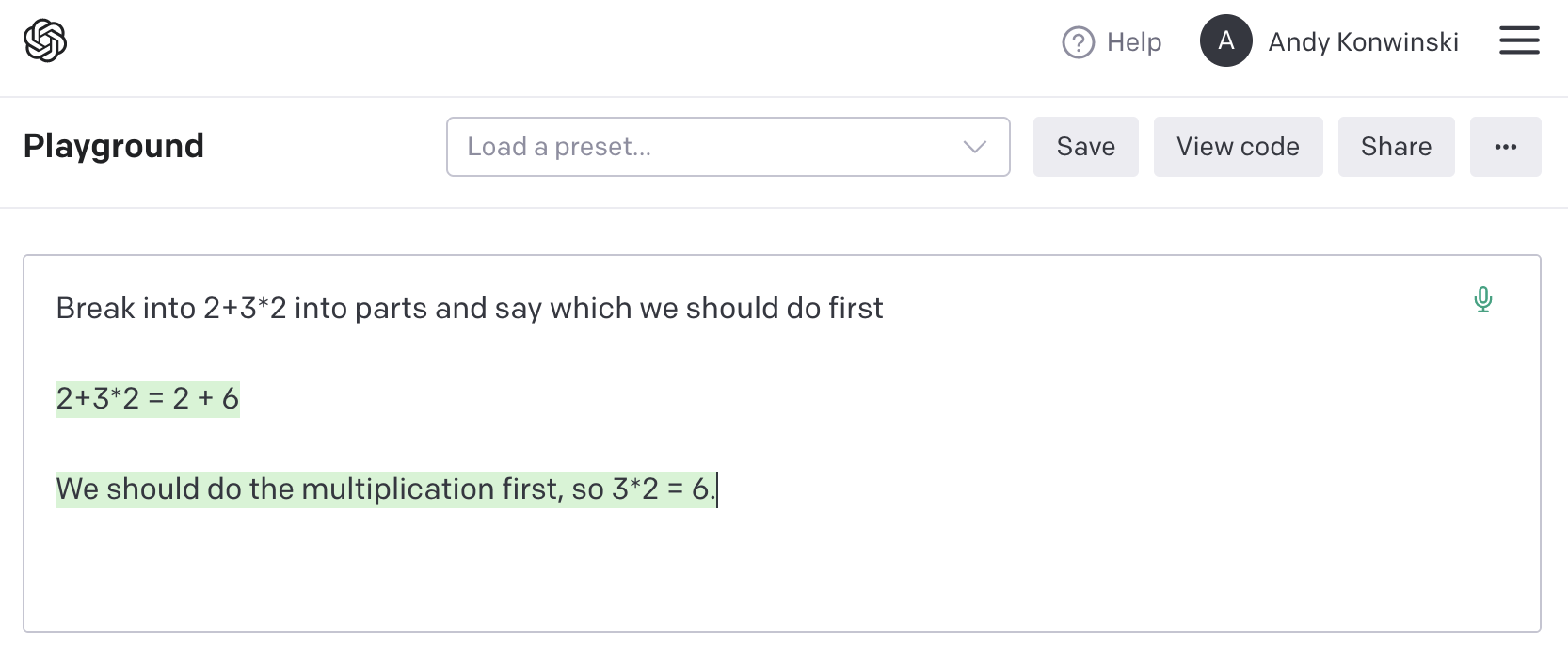Building off of this, we can write “recursive” LLM prompts that utilize facts/rules the model has memorized to take repeated steps towards a solution.

In the case of our original recursive Fibonacci LLM prompt above, this part…

Output this paragraph but with updated variables to compute the next step of the Fibbonaci sequence.

… utilizes the fact that the model has memorized the Fibonacci sequence itself. However, the model isn’t always right!

## Open problem: handling when the model is wrong

An open challenge in using facts memorized by the model for correct reasoning is that LLMs frequently generate incorrect facts. E.g., with the Fibonacci sequence prompt, sometimes it skips a number entirely, sometimes it produces a number that is off-by-some but then gets the following number(s) correct. For example, at the very end of the screen capture video above (i.e., “response #16”) it prints 2504 but the correct answer is 2584.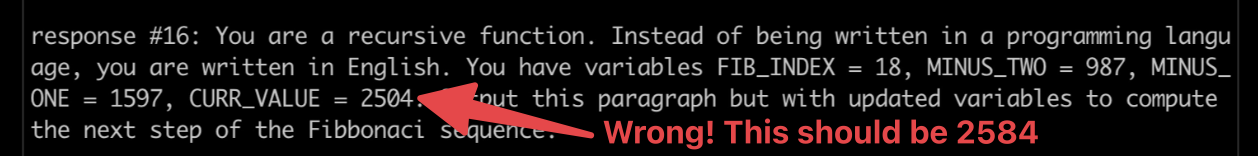This seems to be happening because the model has (1) memorized the Fibonacci sequence, and (2) doesn’t always get it right. We can verify both of these facts by asking the model to return the Fibonacci sequence in a single call…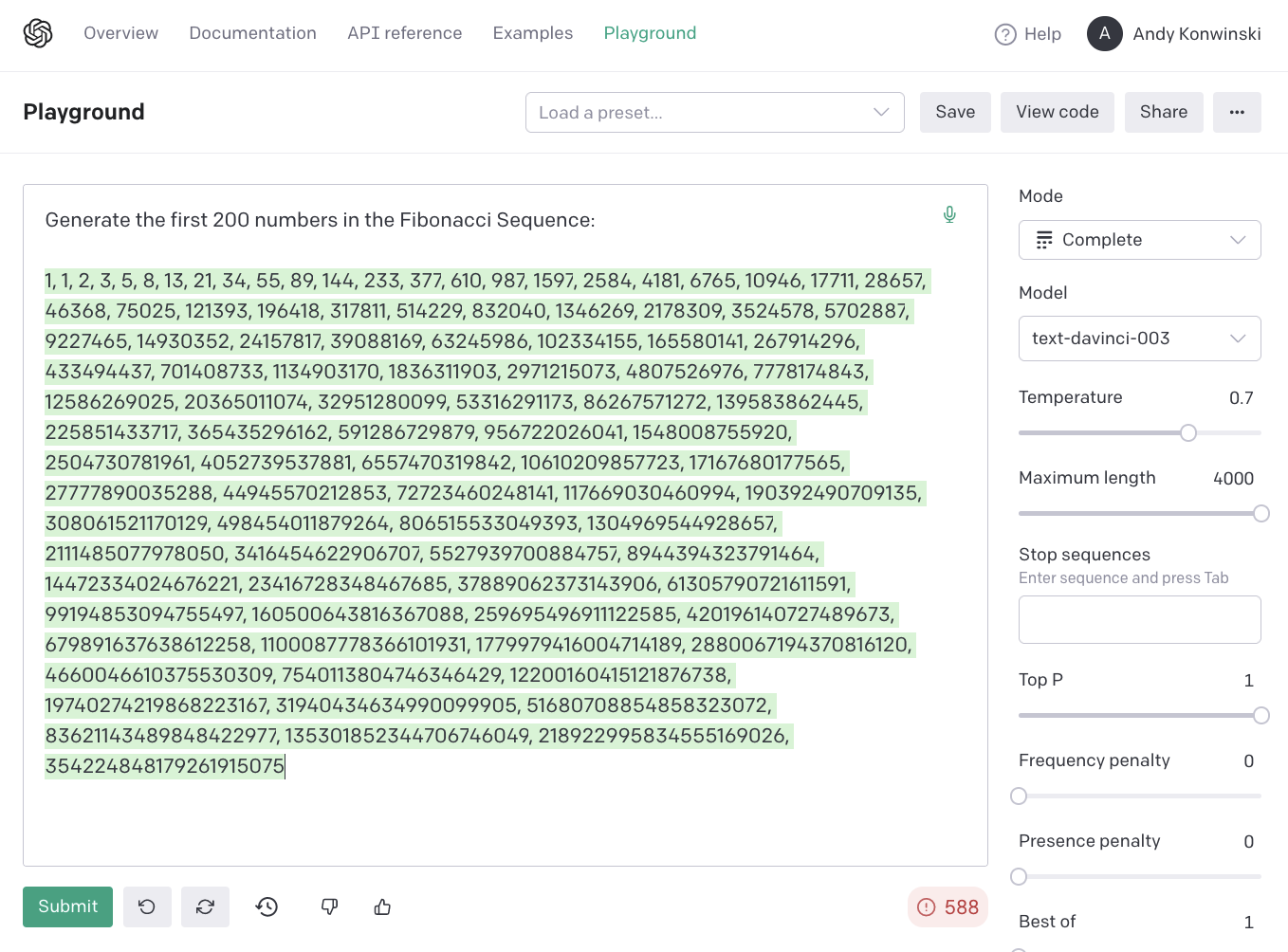…and then comparing what it produces with a the actual sequence (as generate by code):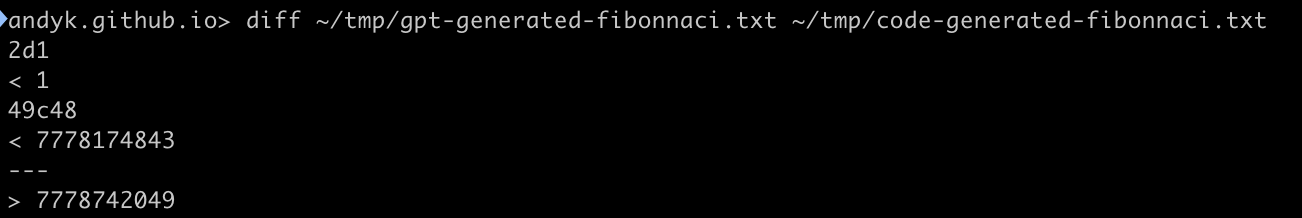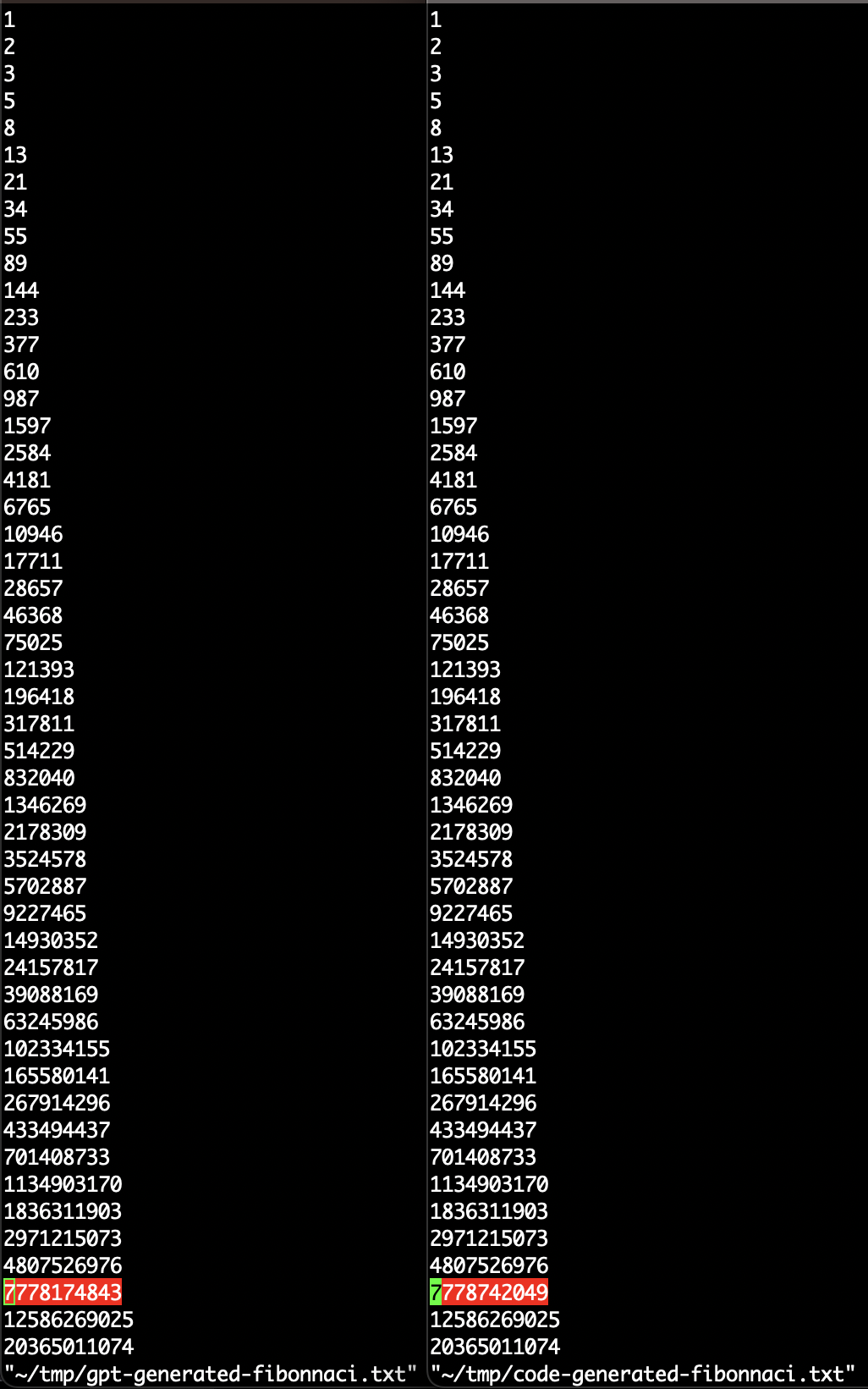One way of handling this is to use more reliable means of looking up facts/knowledge, such as searching Wikipedia, having the model generate and run code, etc. This is an active area of research. If you’re curious about it, check out my list of work on enabling LLMs to do things.

Another idea being explored is having the model fact-check itself. One way to do this is using the model to rephrase the prompt into multiple fact look-ups prompts and then executing all of them and using some voting scheme to decide on an answer with more confidence. This is a technique that has been thoroughly explored in the field of Human Computation research.

Another classic CMU AI research project that extensively explored how human brains leverage memorization and computation is called ACT-R. This project, led by John R. Anderson, explored how humans do math in their head and tried to apply those lessons to their AI agent architecture .

The ACT-R group partnered up with cognitive scientists & neuroscientists and performed FMRIs on students while they were doing math problems.

There are also similarities between this work and ReAct (a contraction of Reasoning + Action) , a “general paradigm” and associated implementation that “…explore[s] the use of LLMs to generate both reasoning traces and task-specific actions…”.

Finally, the idea of recursive prompts was explored in detail in Optimality is the tiger, and agents are its teeth (thanks to mitthrowaway2 on Hackernews for the pointer).

## Beyond Tail-recursion

The examples of recursive LLM prompts considered above are analagous to linear tail recursion, which is essentially iteration implemented via a self-referencing abstraction. In computer science, the abstraction is often implemented in the form of a function that calls itself. In our work here, we are working with a prompt that generates another prompt.

It is even more interesting to explore non-tail recursion, where the abstraction (e.g., the function) performs some transformation of the value returned by its inner call to itself before returning that value to its caller (the next-outer most instance of itself).

To actually compute a simplified value of a non-tail recursive function, a computer system uses a memory stack. In the context of a non-tail recursive prompt, we could try to include the stack as part of the prompt itself.

Here is an example of such a prompt that attempts to recursively compute `factorial(11)`: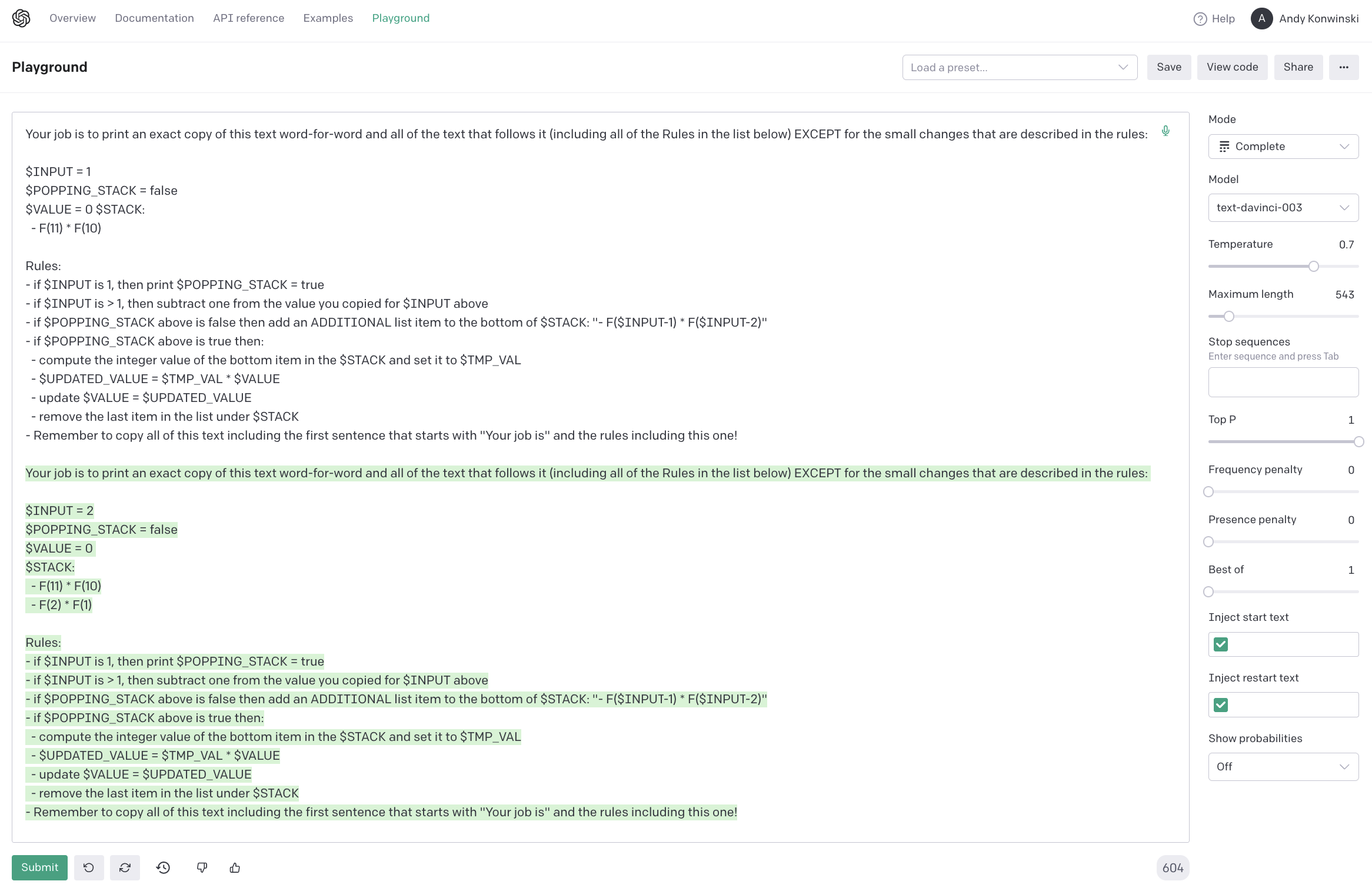You can see the prompt successfully “pushed state” on to the stack. This particular prompt doesn’t yet work after it hits the base case and is supposed to switch modes and pop the stack until it gets to its final value.

Also note that we switched from Fibonacci (which is non-linear when implemented using nontrivial non-tail recursion) to Factorial which is linearly recursive (i.e., only calls itself once). I’m still trying to get this working.

## Acknowledgments

Thanks for Andrew Krioukov, Bill Bultman, Nick Jalbert, Beth Trushkowsky, and Rob Carroll, and the Hackernews community for contributions and feedback!